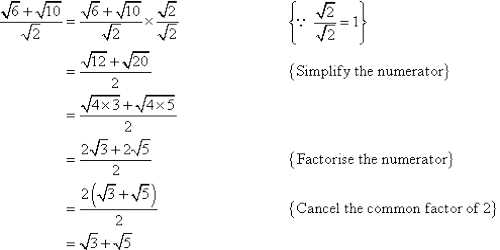Definitions of surds: A root of a positive real quantity is called a surd if its value cannot be exactly determined. It is a number that can’t be simplified to remove a square root (or cube root etc). For example, each of the quantities √3, ∛7, ∜19, (16)^2/5 etc. is a surd.

More Examples:

• √2 (square root of 2) can’t be simplified further so it is a surd
• √4 (square root of 4) CAN be simplified to 2, so it is NOT a surd

Rationalization of Surds

Rationalization is all about moving the surd (√) or complex number to the numerator. Rationalization does not change the value of a number or function but only re-writes it in a more acceptable and most times easier to understand form. “Rationalizing the denominator” is when we move a root (like a square root or cube root) from the bottom of a fraction to the top.

When the denominator of an expression is a surd which can be reduced to an expression with the rational denominator, this process is known as rationalizing the denominator of the surd. In other words, the process of reducing a given surd to a rational form after multiplying it by a suitable surd is known as rationalization.

When the product of two surds is a rational number, then each of the two surds is called rationalizing factor of the other. In other words, if the product of two surds is rational, then each is called a rationalizing factor of the other and each is said to be rationalized by the other.Examples of rationalization of surds:

When the denominator of an expression contains a term with a square root or a number under radical sign, the process of converting into an equivalent expression whose denominator is a rational number is called rationalizing the denominator.

1. For example, the rationalizing factor of √5 is √5 and rationalizing factor of ∛2 is ∛2^2 or ∛4. Since, √5 × √5 = 5 and ∛2 × ∛2^2 = ∛(2 × 2^2) = ∛2^3 = 2
2. (a√z) × (b√z) = (a × b) × (√z × √z) = ab(√z)^2 = abz, which is rational. Therefore, each of the surds a√z and b√z is a rationalizing factor of the other.
3. √5 × 2√5 = 2 × (√5)^2 = 3 × 5 = 15, which is rational. Therefore, each of the surds √5 and 2√5 is a rationalizing factor of the other.
4. (√a + √b) × (√a – √b) = (√a)^2 – (√b)^2 = a – b, which is rational. Therefore, each of the surds (√a + √b) and (√a – √b) is a rationalizing factor of the other.
5. (x√a + y√b) × (x√a – y√b) = (x√a)^2 – (y√b)^2 = ax – by, which is rational. Therefore, each of the surds (x√a + y√b) and (x√a – y√b) is a rationalizing factor of the other.
6. (4√7 + √3) × (4√7 – √3) = (4√7)^2 – (√3)^2 = 112 – 3 = 109, which is rational. Therefore, each of the surd factors (4√7 + √3) and (4√7 – √3) is a rationalizing factor of the other.
7. Also rationalizing factor of ∛(ab^2c^2) is ∛(a^2bc) because ∛(ab^2c^2) × ∛(a^2bc) = abc.

Find the rationalizing factor of (√x – y)

Solution:

Let, √x = x^1/2 = a and ∛y = y^1/3 = b.

Now, the order of the surds √x and ∛y are 2 and 3 respectively and the L.C.M. of 2 and 3 is 6.

Therefore,

a^6 = (x^1/2)^6 = x^3 and b^6 = (y^1/3)^6 = y^2.

Therefore, a^6 and b^6 both are rational and as such (a^6 – b^6) is also rational.

Now, a^6 – b^6 = (a – b)(a^5 + a^4b + a^3b^2 + a^2b^3 + ab^4 + b^5)

Therefore the rationalizing factor of (a – b) = (√x – ∛y) is (a^5 + a^4b + a^3b^2 + a^2b^3 + ab^4 + b^5) = x^5/2 + x^2y^1/3 + x^3/2y^2/3 + xy + x^1/2y^4/3 + y^5/3.

Information Source;